Electron. J. Differential Equations, Vol. 2019 (2019), No. 102, pp. 1-16.

### Non-trivial solutions of fractional Schrodinger-Poisson systems with sum of periodic and vanishing potentials Mingzhu Yu, Haibo Chen

Abstract:
We consider the fractional Schrodinger-Poisson system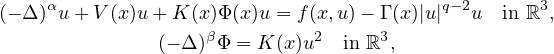where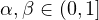,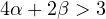,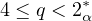, K(x),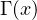and f(x,u) are periodic in x, V is coercive or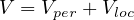is a sum of a periodic potential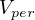and a localized potential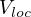. If f has the subcritical growth, but higher than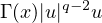, we establish the existence and nonexistence of ground state solutions are dependent on the sign of. Moreover, we prove that such a problem admits infinitely many pairs of geometrically distinct solutions provided that V is periodic and f is odd in u. Finally, we investigate the existence of ground state solutions in the case of coercive potential V.

Submitted March 22, 2018. Published September 4, 2019.
Math Subject Classifications: 35B38, 35G99.
Key Words: Fractional Schrodinger-Poisson system; coercive potential; periodic and localized potential; Nehari manifold; variational method.

Show me the PDF file (384 KB), TEX file for this article.Mingzhu Yu School of Mathematics and Statistics Central South University Changsha, 410083 Hunan, China email: yumz_math@csu.edu.cn Haibo Chen School of Mathematics and Statistics Central South University Changsha, 410083 Hunan, China email: math_chb@163.com